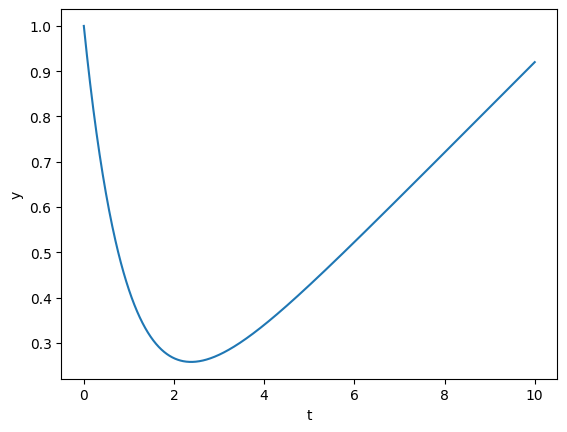# Solving an ODE with a forcing term¤

This example demonstrates how to incorporate an external forcing term into the solve. This is really simple: just evaluate it as part of the vector field like anything else.

This example is available as a Jupyter notebook here.

import jax
import jax.numpy as jnp
import matplotlib.pyplot as plt
from diffrax import diffeqsolve, ODETerm, SaveAt, Tsit5

def force(t, args):
m, c = args
return m * t + c

def vector_field(t, y, args):
return -y + force(t, args)

@jax.jit
def solve(y0, args):
term = ODETerm(vector_field)
solver = Tsit5()
t0 = 0
t1 = 10
dt0 = 0.1
saveat = SaveAt(ts=jnp.linspace(t0, t1, 1000))
sol = diffeqsolve(term, solver, t0, t1, dt0, y0, args=args, saveat=saveat)
return sol

y0 = 1.0
args = (0.1, 0.02)
sol = solve(y0, args)

plt.plot(sol.ts, sol.ys)
plt.xlabel("t")
plt.ylabel("y")
plt.show()Now let's consider a more complicated example: the forcing term is an interpolation, and what's more we would like to differentiate with respect to the values we are interpolating.

from diffrax import backward_hermite_coefficients, CubicInterpolation

def vector_field2(t, y, interp):
return -y + interp.evaluate(t)

@jax.jit
def solve(points):
t0 = 0
t1 = 10
ts = jnp.linspace(t0, t1, len(points))
coeffs = backward_hermite_coefficients(ts, points)
interp = CubicInterpolation(ts, coeffs)
term = ODETerm(vector_field2)
solver = Tsit5()
dt0 = 0.1
y0 = 1.0
sol = diffeqsolve(term, solver, t0, t1, dt0, y0, args=interp)
(y1,) = sol.ys
return y1

points = jnp.array([3.0, 0.5, -0.8, 1.8])